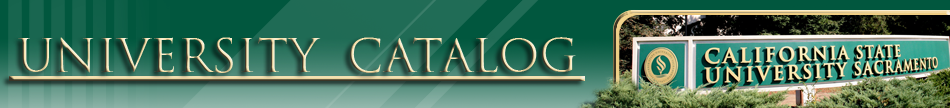STAT 1. Introduction to Statistics. Descriptive statistics, basic concepts of probability and sampling with the aim of introducing fundamental notions and techniques of statistical inference. Prerequisite: MATH 9 or three years of high school mathematics which includes two years of algebra and one year of geometry; completion of ELM requirement and the Intermediate Algebra Diagnostic Test. Graded: Graded Student. Units: 3.0
STAT 10A. Introductory Statistics with Developmental Mathematics. Data analysis process and the concept of randomness. Descriptive statistics: Summarizing the data distribution graphically and numerically. Reasoning about bivariate numerical data; linear correlation and regression. Linear, quadratic and exponential functions as a way of modeling a correspondence between two variables. Reasoning about bivariate categorical data. Basic concepts of probability. Discrete and random variables. Binomial distribution. Introduction to normal distribution. Lecture three hours. Prerequisite: A minimum ELM score of 44, and being placed in Learning Skills Program for one semester of developmental mathematics. Graded: Graded Student. Units: 3.0
STAT 10B. Introductory Statitstics with Developmental Mathematics. Continuation of STAT 10A. Continuous random variables. The normal distribution. Sampling distributions and the central limit theorem. Confidence intervals and tests of hypotheses for means, proportions, difference in means and difference in proportions. Chi-squared tests. Lecture three hours. Prerequisite: Successful completion of STAT 10A with a minimum "C-" grade or better. Graded: Graded Student. Units: 3.0
STAT 50. Introduction to Probability and Statistics. Sample spaces, combinatorics, and random variables. Density and distribution functions. Expectation, variance, and covariance. The binomial, uniform, poisson, negative binomial, hypergeometric, exponential, gamma, beta, and normal distributions. Sampling distributions, estimation, and hypothesis tests. Students are given periodic writing assignments which encourage them to think through concepts of the course. Prerequisite: MATH 26A, MATH 30, or appropriate high school based AP credit. Graded: Graded Student. Units: 4.0
STAT 96. Experimental Offerings in Statistics. When there is a demand from a sufficient number of qualified students, one of the staff will conduct a seminar on some topic in statistics. Graded: Graded Student. Units: 1.0 - 6.0.
STAT 103. Intermediate Statistics. Review of hypothesis testing --one sample. Hypothesis testing --two sample, variance. Regression and correlation. Analysis of variance including two-way. Analysis of categorical data. Non-parametric tests, goodness of fit, and tests for randomness. Prerequisite: STAT 50 or instructor consent Graded: Graded Student. Units: 3.0
STAT 115A. Introduction to Probability Theory. Probability axioms, discrete and continuous random variables, functions of random variables, joint densities, expectation, moment generating functions. Chebyshev's inequality, transformations, weak law of large numbers, central limit theorem. Prerequisite: MATH 31 and either STAT 1 or STAT 50 Graded: Graded Student. Units: 3.0
STAT 115B. Introduction to Mathematical Statistics. Point Estimation, interval estimation, hypothesis testing, the multivariate normal distribution, non-parametric tests. Prerequisite: STAT 115A. Graded: Graded Student. Units: 3.0
STAT 155. Introduction to Techniques of Operations Research. Formulation and analysis of mathematical models with emphasis on real systems applications. Introduction to Queueing theory and Markov Processes for application. Prerequisite: MATH 31; STAT 50, STAT 103, or STAT 115A; MATH 31 may be taken concurrently. Graded: Graded Student. Units: 3.0
STAT 196J. Statistical Computing. Computer methods for accessing, transforming, summarizing, graphing, and making statistical inferences from data. Both a command-line statistical software package such as R and menu-driven package such as SAS will be used. Students will learn to apply computer methods to solve problems selected from the areas of modeling, simulation, inference and statistical learning. The intent of this course is to provide students with the software skills needed for statistical work in industry or academia. Prerequisite: STAT 1 or STAT 50, and MATH 26A or MATH 30 Graded: Graded Student. Units: 3.0
STAT 199. Special Problems. Individual projects or directed reading. Open only to students who appear competent to carry on individual work. Admission to this course requires approval of the instructor in addition to the approval of the advisor and the Department Chair. Graded: Graded (CR/NC Available). Units: 1.0 - 6.0.
STAT 215A. Introduction to Mathematical Statistics. Probability measure, conditional probability and independence, random variables, characteristic and moment-generating functions, modes of convergence. Prerequisite: STAT 115A, STAT 115B; MATH 134 is recommended. Graded: Graded Student. Units: 3.0
STAT 215B. Topics in Introduction to Mathematical Statistics. Continuation of STAT 215A with topics selected from: Statistical Inference, Estimation Theory, Testing Hypotheses, Linear Models, Nonparametric Methods, Multivariate Analysis, Computer intensive methods in Statistics and Sampling Theory. Note: May be taken twice with approval of Graduate Coordinator. Prerequisite: STAT 215A. Graded: Graded Student. Units: 3.0
STAT 299. Special Problems. Any properly qualified student who wishes to pursue a problem may do so if the proposed subject is acceptable to the department committee, the supervising instructor and the student's advisor. Graded: Graded Student. Units: 1.0 - 6.0.